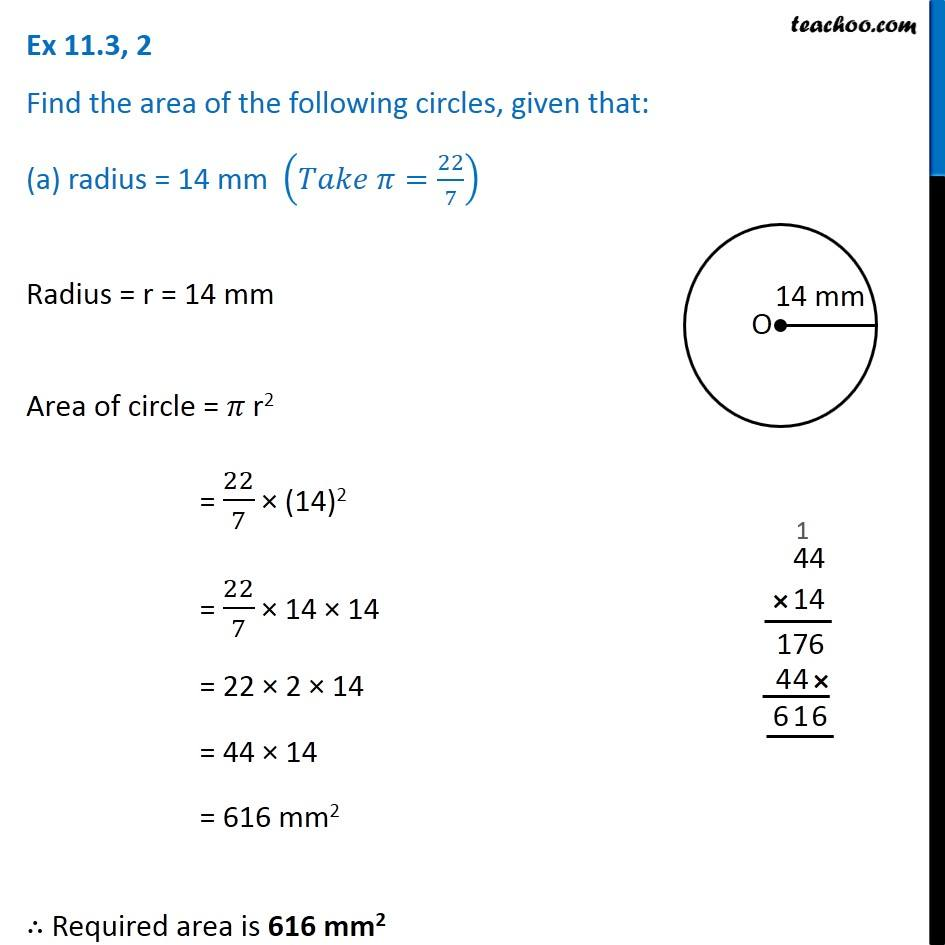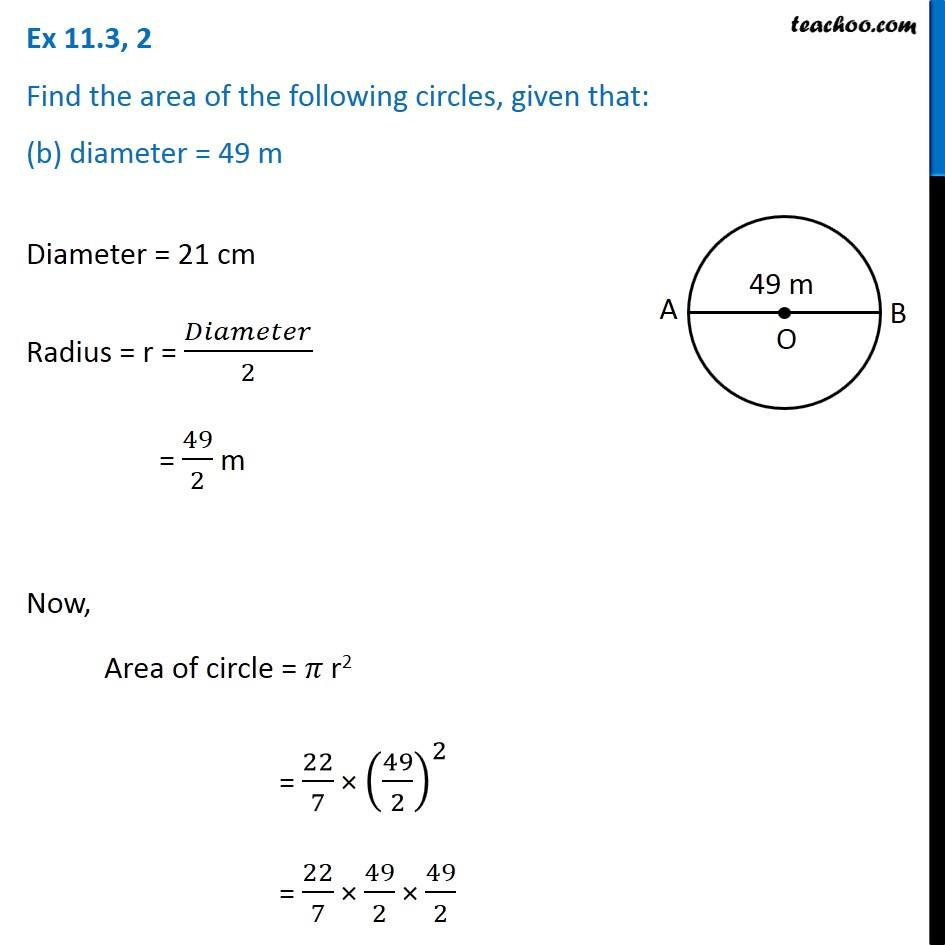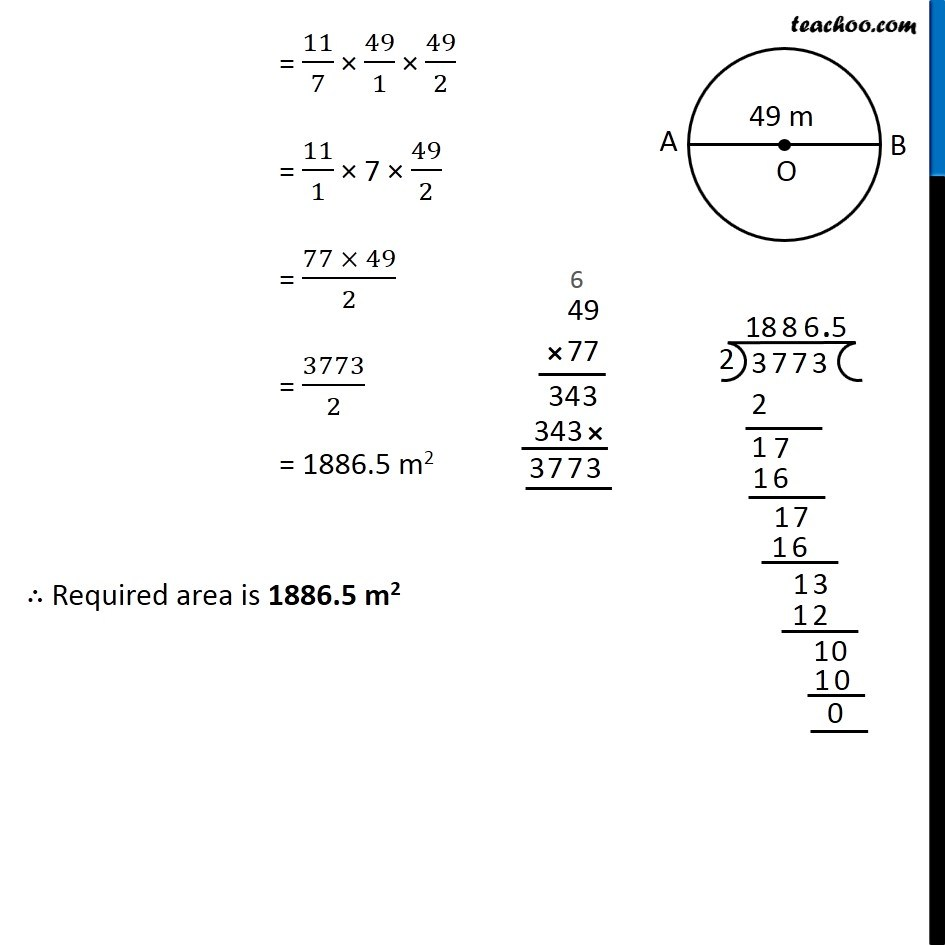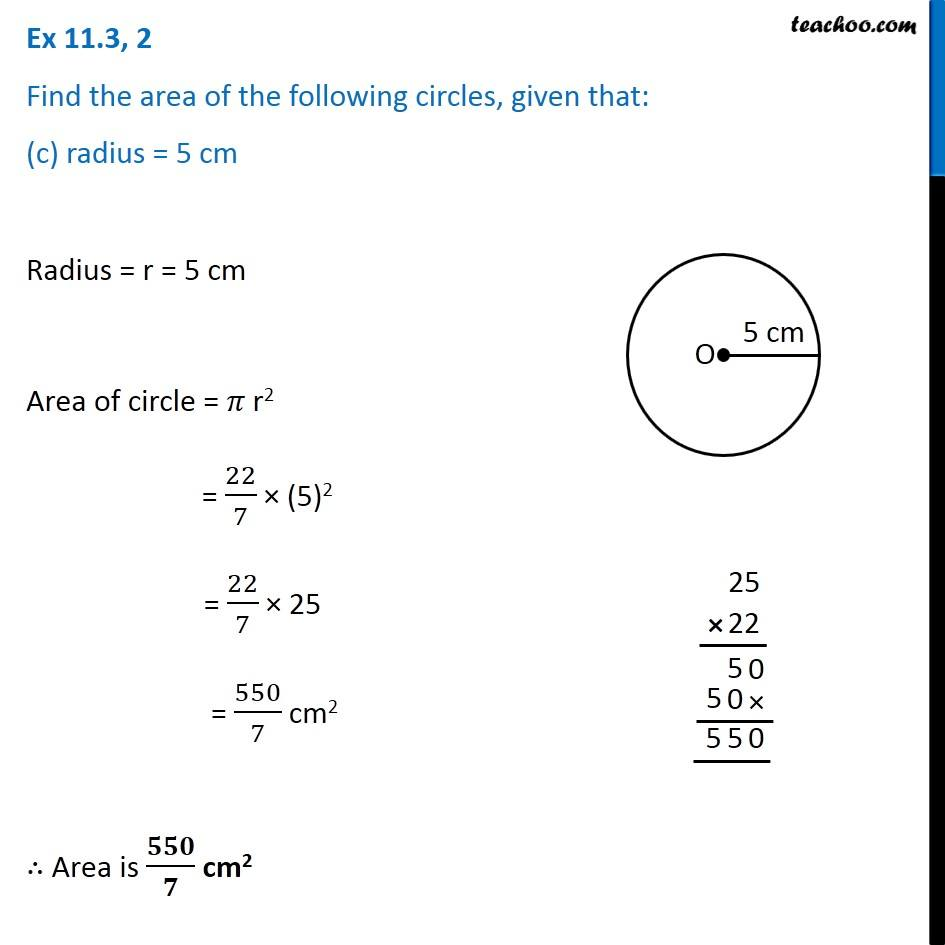Subscribe to our Youtube Channel - https://you.tube/teachoo

1. Chapter 11 Class 7 Perimeter and Area
2. Serial order wise
3. Ex 11.3

Transcript

Ex 11.3, 2 Find the area of the following circles, given that: (a) radius = 14 mm (𝑇𝑎𝑘𝑒 𝜋=22/7) Radius = r = 14 mm Area of circle = 𝜋 r2 = 22/7 × (14)2 = 22/7 × 14 × 14 = 22 × 2 × 14 = 44 × 14 = 616 mm2 ∴ Required area is 616 mm2 Ex 11.3, 2 Find the area of the following circles, given that: (b) diameter = 49 m Diameter = 21 cm Radius = r = 𝐷𝑖𝑎𝑚𝑒𝑡𝑒𝑟/2 = 49/2 m Now, Area of circle = 𝜋 r2 = 22/7 × (49/2)^2 = 22/7 × 49/2 × 49/2 = 11/7 × 49/1 × 49/2 = 11/1 × 7 × 49/2 = (77 × 49)/2 = 3773/2 = 1886.5 m2 ∴ Required area is 1886.5 m2 Ex 11.3, 2 Find the area of the following circles, given that: (c) radius = 5 cm Radius = r = 5 cm Area of circle = 𝜋 r2 = 22/7 × (5)2 = 22/7 × 25 = 550/7 cm2 ∴ Area is 𝟓𝟓𝟎/𝟕 cm2

Ex 11.3